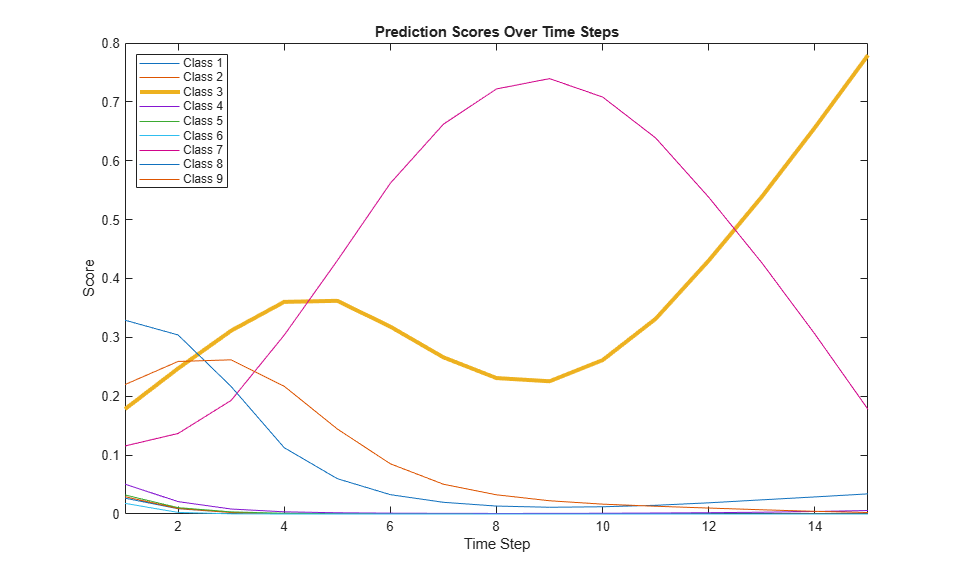# Predict and Update Network State in Simulink

This example shows how to predict responses for a trained recurrent neural network in Simulink® by using the `Stateful Predict` block. This example uses a pretrained long short-term memory (LSTM) network.

Load `JapaneseVowelsNet`, a pretrained long short-term memory (LSTM) network trained on the Japanese Vowels data set as described in  and . This network was trained on the sequences sorted by sequence length with a mini-batch size of 27.

```load JapaneseVowelsNet ```

View the network architecture.

```analyzeNetwork(net); ```

Load the Japanese Vowels test data. `XTest` is a cell array containing 370 sequences of dimension 12 of varying length. `TTest` is a categorical vector of labels "1","2",..."9", which correspond to the nine speakers.

Create a timetable array `simin` with time-stamped rows and repeated copies of `X`.

```[XTest,TTest] = japaneseVowelsTestData; X = XTest{94}; numTimeSteps = size(X,2); simin = timetable(repmat(X,1,4)','TimeStep',seconds(0.2)); ```

### Simulink Model for Predicting Responses

The Simulink model for predicting responses contains a `Stateful Predict` block to predict the scores and `From Workspace` block to load the input data sequence over the time steps.

To reset the state of recurrent neural network to its initial state during simulation, place the `Stateful Predict` block inside a `Resettable Subsystem` and use the `Reset` control signal as trigger.

```open_system('StatefulPredictExample'); ```### Configure Model for Simulation

Set the model configuration parameters for the `Stateful Predict` block.

```set_param('StatefulPredictExample/Stateful Predict','NetworkFilePath','JapaneseVowelsNet.mat'); set_param('StatefulPredictExample', 'SimulationMode', 'Normal'); ```

### Run the Simulation

To compute responses for the `JapaneseVowelsNet` network, run the simulation. The prediction scores are saved in the MATLAB® workspace.

```out = sim('StatefulPredictExample'); ```

Plot the prediction scores. The plot shows how the prediction scores change between time steps.

```scores = squeeze(out.yPred.Data(:,:,1:numTimeSteps)); classNames = string(net.Layers(end).Classes); figure lines = plot(scores'); xlim([1 numTimeSteps]) legend("Class " + classNames,'Location','northwest') xlabel("Time Step") ylabel("Score") title("Prediction Scores Over Time Steps") ```Highlight the prediction scores over time steps for the correct class.

```trueLabel = TTest(94); lines(trueLabel).LineWidth = 3; ```Display the final time step prediction in a bar chart.

```figure bar(scores(:,end)) title("Final Prediction Scores") xlabel("Class") ylabel("Score") ```M. Kudo, J. Toyama, and M. Shimbo. "Multidimensional Curve Classification Using Passing-Through Regions." Pattern Recognition Letters. Vol. 20, No. 11–13, pages 1103–1111.

 UCI Machine Learning Repository: Japanese Vowels Dataset. https://archive.ics.uci.edu/ml/datasets/Japanese+Vowels